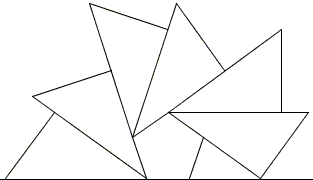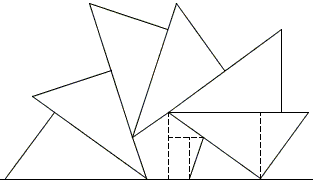# Out of Pentagon Sangaku

Many sangaku problems include circle and ellipses, but quite a few do not. An elegant one [Fakagawa & Pedoe, p. 49] with a rather computational solution is presented below.6 congruent right triangles fan out along the sides of a regular pentagon of side a. Find the length of the hypotenuse t of these triangles in terms of a.

This sangaku appears on a extant tablet in the Miyagai prefecture. Unless there is a misprint, [Fakagawa & Pedoe, p. 134] dates the tablet from 1912.

Solution

### References

1. H. Fukagawa, D. Pedoe, Japanese Temple Geometry Problems, The Charles Babbage Research Center, Winnipeg, 1989

Write to:

Charles Babbage Research Center
P.O. Box 272, St. Norbert Postal Station
Winnipeg, MB6 congruent right triangles fan out along the sides of a regular pentagon of side a. Find the length of the hypotenuse t of these triangles in terms of a.

### Solution

We can imagine a couple of right triangles with sides computable from the Pythagorean theorem that combine into the altitude of one of the congruent triangles:Given that the internal angle of a regular pentagon is 108°, one of the triangles has angles 36°- 54°- 90°, the other 18°- 72°- 90°.

The altitude in question equals

h = a·(sin(36°) + sin(72°)).

The fan forming triangles are also of the 36°- 54°- 90° variety since two of their smallest angles supplement 108°. In such a triangle the hypotenuse t is expressable in terms of the altitude:

t = h·(tan(36°) + tan(54°)).

Combining the two we get

t = a·(tan(36°) + tan(54°))·(sin(36°) + sin(72°)).

The solution t = a·(1 + 5) given by [Fakagawa & Pedoe] tells us that the expression for t, if correct, is amenable to a simplification effort.

Let's denote c = cos(36°) and s = sin(36°). By the Pythagorean theorem c2 + s2 = 1. In addition, tan(36°) = s/c, and tan(54°) = cot(36°) = c/s, since 36° and 54° are complementary angles. Also, sin(72°) = 2sc. We see that t can be written as

 t = a·(tan(36°) + tan(54°))·(sin(36°) + sin(72°)) = a·(s/c + c/s)·(s + 2sc) = a·s/sc·(s2 + c2)·(1 + 2c) = a·(1 + 2c)/c

This must be transformed further taking into account that c = (1 + 5)/4. To continue,

 t = a·(1 + 2c)/c = a·(1 + (1 + √5)/2) / (1 + √5)·4 = a·(3 + √5)) / (1 + √5)·2 = a·(3 + √5))·(-1 + √5) / 2 = a·(-3 + 5 - √5 + 3√5) / 2 = a·(2 + 2√5) / 2 = a·(1 + √5).## Sangaku